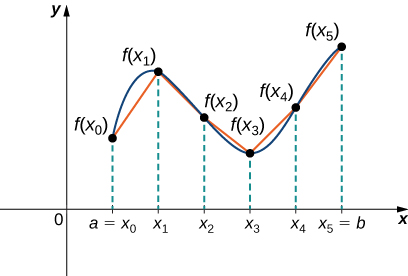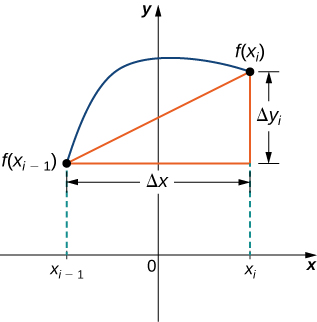# 6.4 Arc length of a curve and surface area

 Page 1 / 8
• Determine the length of a curve, $y=f\left(x\right),$ between two points.
• Determine the length of a curve, $x=g\left(y\right),$ between two points.
• Find the surface area of a solid of revolution.

In this section, we use definite integrals to find the arc length of a curve. We can think of arc length    as the distance you would travel if you were walking along the path of the curve. Many real-world applications involve arc length. If a rocket is launched along a parabolic path, we might want to know how far the rocket travels. Or, if a curve on a map represents a road, we might want to know how far we have to drive to reach our destination.

We begin by calculating the arc length of curves defined as functions of $x,$ then we examine the same process for curves defined as functions of $y.$ (The process is identical, with the roles of $x$ and $y$ reversed.) The techniques we use to find arc length can be extended to find the surface area of a surface of revolution, and we close the section with an examination of this concept.

## Arc length of the curve y = f ( x )

In previous applications of integration, we required the function $f\left(x\right)$ to be integrable, or at most continuous. However, for calculating arc length we have a more stringent requirement for $f\left(x\right).$ Here, we require $f\left(x\right)$ to be differentiable, and furthermore we require its derivative, ${f}^{\prime }\left(x\right),$ to be continuous. Functions like this, which have continuous derivatives, are called smooth . (This property comes up again in later chapters.)

Let $f\left(x\right)$ be a smooth function defined over $\left[a,b\right].$ We want to calculate the length of the curve from the point $\left(a,f\left(a\right)\right)$ to the point $\left(b,f\left(b\right)\right).$ We start by using line segments to approximate the length of the curve. For $i=0,\phantom{\rule{0.2em}{0ex}}1,2\text{,…},n,$ let $P=\left\{{x}_{i}\right\}$ be a regular partition of $\left[a,b\right].$ Then, for $i=1,2\text{,…},n,$ construct a line segment from the point $\left({x}_{i-1},f\left({x}_{i-1}\right)\right)$ to the point $\left({x}_{i},f\left({x}_{i}\right)\right).$ Although it might seem logical to use either horizontal or vertical line segments, we want our line segments to approximate the curve as closely as possible. [link] depicts this construct for $n=5.$We can approximate the length of a curve by adding line segments.

To help us find the length of each line segment, we look at the change in vertical distance as well as the change in horizontal distance over each interval. Because we have used a regular partition, the change in horizontal distance over each interval is given by $\text{Δ}x.$ The change in vertical distance varies from interval to interval, though, so we use $\text{Δ}{y}_{i}=f\left({x}_{i}\right)-f\left({x}_{i-1}\right)$ to represent the change in vertical distance over the interval $\left[{x}_{i-1},{x}_{i}\right],$ as shown in [link] . Note that some (or all) $\text{Δ}{y}_{i}$ may be negative.A representative line segment approximates the curve over the interval [ x i − 1 , x i ] .

By the Pythagorean theorem, the length of the line segment is $\sqrt{{\left(\text{Δ}x\right)}^{2}+{\left(\text{Δ}{y}_{i}\right)}^{2}}.$ We can also write this as $\text{Δ}x\sqrt{1+{\left(\left(\text{Δ}{y}_{i}\right)\text{/}\left(\text{Δ}x\right)\right)}^{2}}.$ Now, by the Mean Value Theorem, there is a point ${x}_{i}^{*}\in \left[{x}_{i-1},{x}_{i}\right]$ such that ${f}^{\prime }\left({x}_{i}^{*}\right)=\left(\text{Δ}{y}_{i}\right)\text{/}\left(\text{Δ}x\right).$ Then the length of the line segment is given by $\text{Δ}x\sqrt{1+{\left[{f}^{\prime }\left({x}_{i}^{*}\right)\right]}^{2}}.$ Adding up the lengths of all the line segments, we get

$\text{Arc Length}\phantom{\rule{0.2em}{0ex}}\approx \sum _{i=1}^{n}\sqrt{1+{\left[{f}^{\prime }\left({x}_{i}^{*}\right)\right]}^{2}}\phantom{\rule{0.2em}{0ex}}\text{Δ}x.$

This is a Riemann sum. Taking the limit as $n\to \infty ,$ we have

#### Questions & Answers

Find the derivative of g(x)=−3.
Abdullah Reply
any genius online ? I need help!!
Guzorochi Reply
how can i help you?
Pina
need to learn polynomial
Zakariya
i will teach...
nandu
I'm waiting
Zakariya
plz help me in question
Abish
evaluate the following computation (x³-8/x-2)
Murtala Reply
teach me how to solve the first law of calculus.
Uncle Reply
what is differentiation
Ibrahim Reply
f(x) = x-2 g(x) = 3x + 5 fog(x)? f(x)/g(x)
Naufal Reply
fog(x)= f(g(x)) = x-2 = 3x+5-2 = 3x+3 f(x)/g(x)= x-2/3x+5
diron
pweding paturo nsa calculus?
jimmy
how to use fundamental theorem to solve exponential
JULIA Reply
find the bounded area of the parabola y^2=4x and y=16x
Omar Reply
what is absolute value means?
Geo Reply
Chicken nuggets
Hugh
🐔
MM
🐔🦃 nuggets
MM
(mathematics) For a complex number a+bi, the principal square root of the sum of the squares of its real and imaginary parts, √a2+b2 . Denoted by | |. The absolute value |x| of a real number x is √x2 , which is equal to x if x is non-negative, and −x if x is negative.
Ismael
find integration of loge x
Game Reply
find the volume of a solid about the y-axis, x=0, x=1, y=0, y=7+x^3
Godwin Reply
how does this work
Brad Reply
Can calculus give the answers as same as other methods give in basic classes while solving the numericals?
Cosmos Reply
log tan (x/4+x/2)
Rohan
please answer
Rohan
y=(x^2 + 3x).(eipix)
Claudia
is this a answer
Ismael
A Function F(X)=Sinx+cosx is odd or even?
WIZARD Reply
neither
David
Neither
Lovuyiso
f(x)=1/1+x^2 |=[-3,1]
Yuliana Reply
apa itu?
fauzi

### Read also:

#### Get Jobilize Job Search Mobile App in your pocket Now!

Source:  OpenStax, Calculus volume 1. OpenStax CNX. Feb 05, 2016 Download for free at http://cnx.org/content/col11964/1.2
Google Play and the Google Play logo are trademarks of Google Inc.

Notification Switch

Would you like to follow the 'Calculus volume 1' conversation and receive update notifications?By Sarah WarrenBy OpenStaxBy OpenStaxBy Stephen VoronBy Yacoub JayoghliBy Jill ZerressenBy Janet ForresterBy OpenStaxBy Tod McGrathBy Richley Crapo